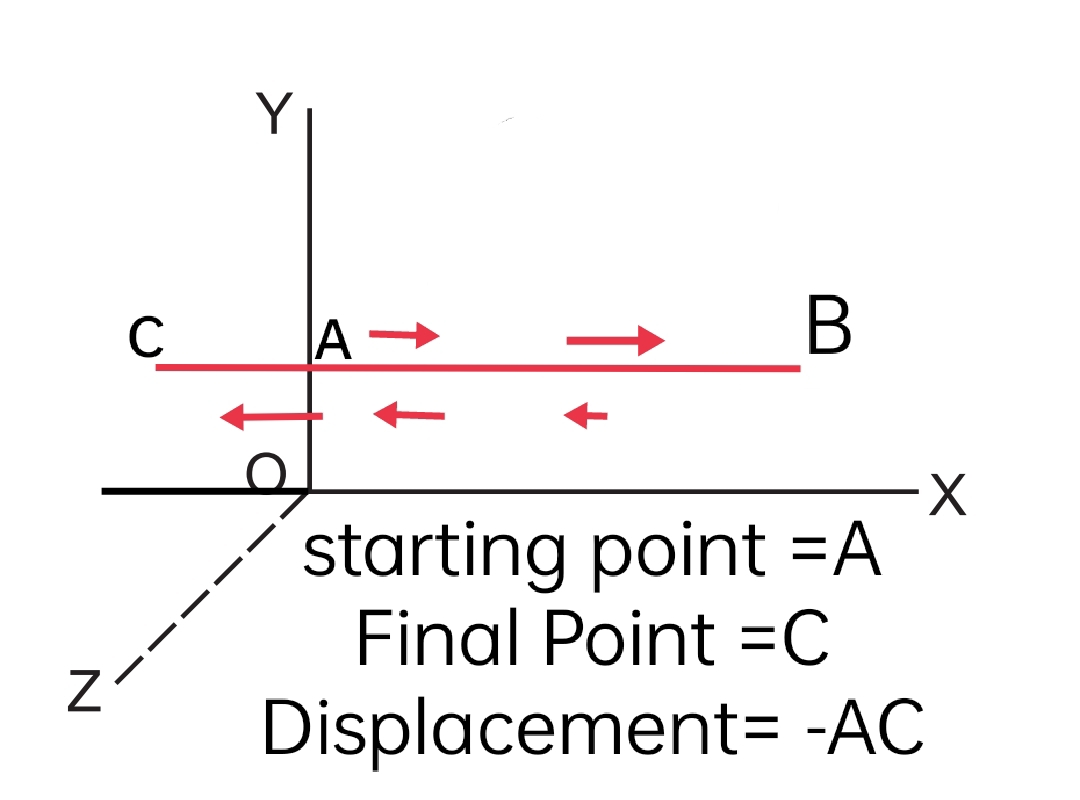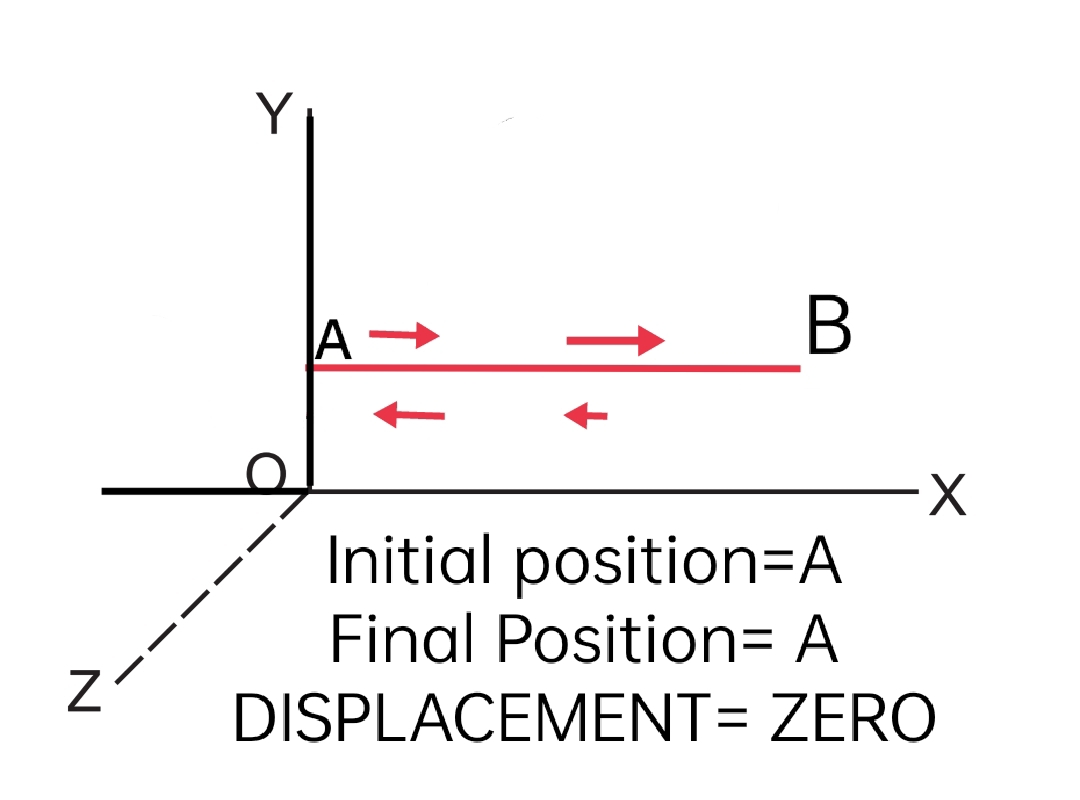# [physics] Distance and Displacement { types and examples}

## Distance

### Best Definition with example:

Distance is the length of the original path covered by a moving object in a given time interval. for example, suppose an object is at point A at time t1 and at point B at time t2. during the time interval t1 to t2, the object moves along the path ACB. The length of the path ACB is called Distance.## Displacement

### Best Definition with example:

Displacement is the shortest distance covered by a body in a particular direction. for example, if an object is at point A at time t1 and at point B at time t2. during the time interval t1 to t2, the object moves along the path ACB. the shortest length from A to B called Displacement.

in simple words, Displacement is the range from the initial position to the final position covered by an object.

#### Important Key points regarding Distance and Displacement:

• Distance is a scalar quantity whereas Displacement is a vector quantity but both having the same Unit i.e. meter/metre.
• Displacement may be positive, negative, or zero whereas distance is always positive.
• The magnitude of displacement is less than or equal to the magnitude of distance.

## Difference between Distance and Displacement:

The difference between Distance and Displacement is that Distance is a Scalar quantity whereas Displacement is a vector quantity. Displacement can be positive, negative, or zero but Distance is always positive.

## Types of Displacement:

There are three types of displacement.

1. Positive Displacement
1. Negative Displacement
1. Zero Displacement

Here are the explanations of three kinds of displacement in physics

## Positive Displacement:When an object covers a certain distance from its initial position to its final position in the direction of the positive axis from the origin is said to be a Positive Displacement. for example, suppose an object is at its initial position A moves to point B. then AB is the positive Displacement.

## Negative Displacement:A Displacement is considered to be Negative when an object from its origin covers a certain distance towards the negative axis. for example, if an Object moves to point B from its initial Position A and then after returning to its initial position A, it travels to point C which is on the negative Axis or in the backward direction of the object then the Displacement AC is said to be Negative Displacement.

in simple words, when an object covers a distance in the backward direction with reference to its original position is called Negative Displacement.

## Zero Displacement:A zero displacement is that when the initial position of the object remains the same I.e. zero change in the initial position of the particle. for example, suppose an object is at point A travels to point B and then returns to its initial position point A, displacement is said to be Zero.

### Brief note:

The magnitude of the displacement is the length of the straight line joining the initial and the final position. The direction is from the initial to the final position. The displacement has both the magnitude as well as the direction. Further, the displacements add according to the triangle rule of vector addition.

Suppose a particle kept on a table is displaced on the table and at the same time the table is also displaced in the room. The net displacement of the particle in the room is obtained by the vector sum of the two displacements. Thus, displacement is a vector quantity. In contrast, the distance covered has only a magnitude and is thus a scalar quantity.